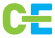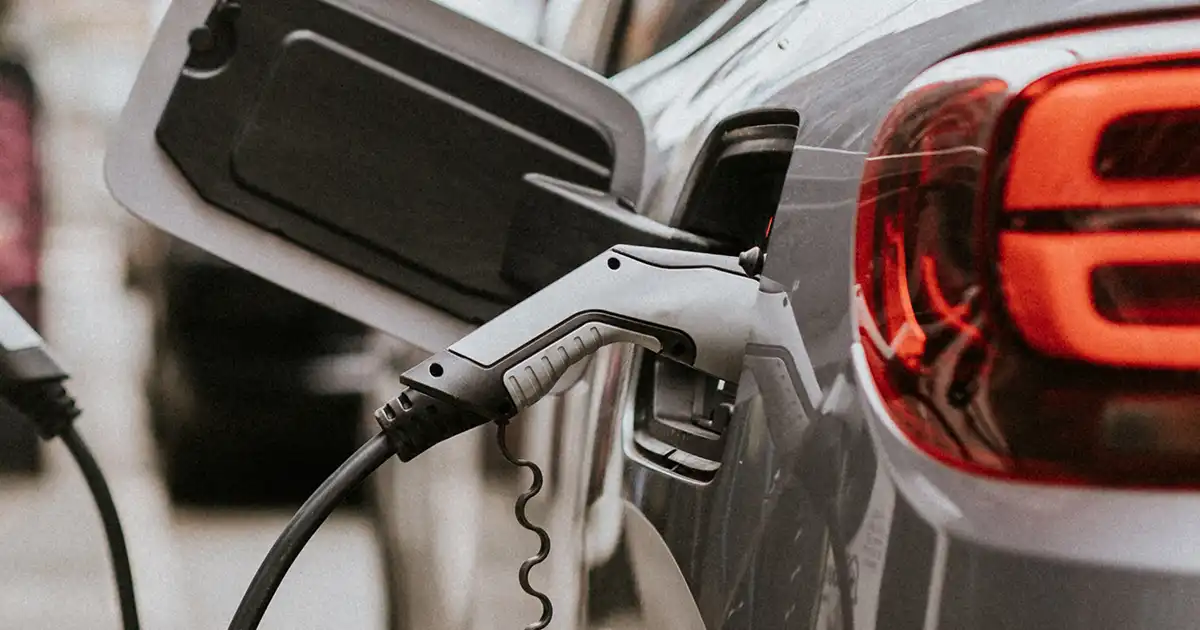# EV Charging: Costs for Home and Fast Charging in India

Introduction:

The shift to electric vehicles (EVs) brings forth the need to understand the intricacies of charging costs—a key factor in the embrace of sustainable mobility. In this comprehensive guide, we explore the cost of charging an electric vehicle in India, considering both home charging and fast charging at public stations. Using a standard electric car as an example, we provide detailed calculations for both scenarios, shedding light on the financial aspects of EV ownership.

The Landscape of EV Charging in India:

Public Charging Stations:

The proliferation of public charging stations marks a pivotal aspect of India’s evolving EV infrastructure. We delve into the convenience and costs associated with utilizing these public charging points, especially those equipped with fast chargers.

Home Charging Infrastructure:

Charging an electric vehicle at home is a convenient option for many EV owners. We explore the types of home charging solutions, from standard wall sockets to dedicated home charging points, and discuss the cost implications.

Calculating the Cost of Charging:

Electricity Rates:

Understanding the electricity rates in your locality is the first step in calculating the cost of charging. Rates may vary, and some regions offer special tariffs for EV charging.

Charging Efficiency:

Different electric vehicles have varying charging efficiencies. Factors such as the efficiency of the charging system and the state of charge of the battery impact the overall energy consumption.

Example Calculation: Charging a Standard Electric Car:

Let’s consider a standard electric car with the following specifications:

• Battery Capacity: 60 kWh
• Energy Consumption Rate: 15 kWh/100 miles
• Charging Efficiency: 90%

Assuming you want to charge the electric car from 20% to 80%, here’s the calculation for both home and fast charging:

Home Charging:

Calculate the Energy Needed:

Energy needed = Battery Capacity * (Final State of Charge – Initial State of Charge)

Energy needed = 60 kWh * (0.8 – 0.2) = 24 kWh

Calculate the Charging Time:

Charging Time = Energy Needed / Charging Power

Assuming a 7 kW charging power for home charging:

Charging Time = 24 kWh / 7 kW ≈ 3.43 hours

Calculate the Cost of Charging:

Cost of Charging = Energy Needed * Electricity Rate

Assuming an average electricity rate of ₹7 per kWh:

Cost of Charging = 24 kWh * ₹7 = ₹168

Fast Charging at Public Station:

Calculate the Charging Time:

Charging Time = Energy Needed / Charging Power

Assuming a fast-charging station with a charging power of 50 kW:

Charging Time = 24 kWh / 50 kW = 0.48 hours (approximately 29 minutes)

Calculate the Cost of Fast Charging:

Cost of Fast Charging = Energy Needed * Charging Rate

Assuming a charging rate of ₹10 per kWh at the fast-charging station:

Cost of Fast Charging = 24 kWh * ₹10 = ₹240

Conclusion:

In conclusion, this guide offers a holistic view of the cost considerations associated with charging an electric vehicle in India, backed by a practical example calculation. Whether utilizing public charging stations with fast chargers or opting for the convenience of home charging, understanding the financial implications is crucial for informed decision-making in the journey toward sustainable and cost-effective mobility. As the EV landscape continues to evolve, embracing the calculations and insights provided herein empowers individuals to navigate the charge and contribute to the green revolution on India’s roads.

## Check out more blogs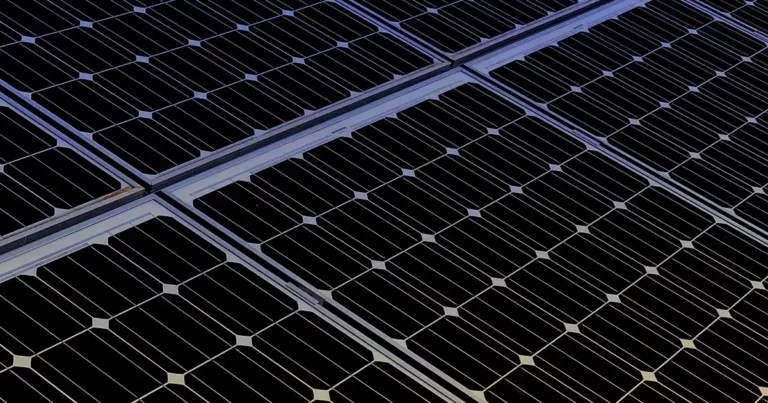A Comprehensive Guide to Solar Panels for Home Use...Solar Energy in Sports Stadiums in India: A Greener...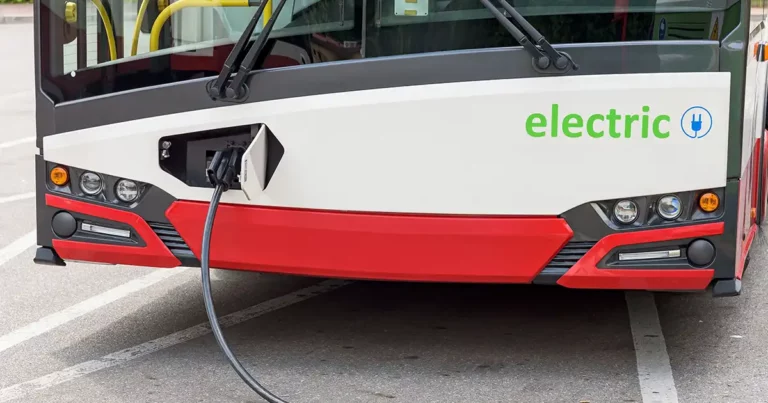Solar-Powered Public Transportation: Case Study in...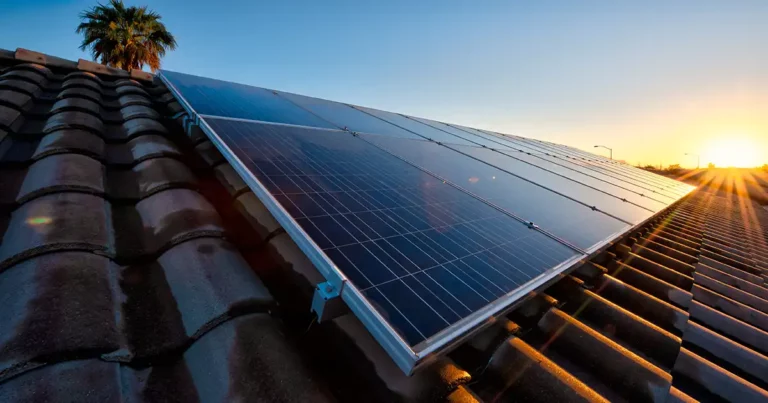The Rise of Mono PERC: A Game-Changer in Solar Energy...Solar Energy and the Fight Against Air Pollution Introduction...